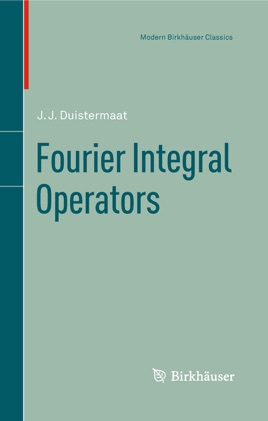• \$84.99

## Publisher Description

This volume is a useful introduction to the subject of Fourier integral operators and is based on the author's classic set of notes. Covering a range of topics from Hörmander’s exposition of the theory, Duistermaat approaches the subject from symplectic geometry and includes applications to hyperbolic equations (= equations of wave type) and oscillatory asymptotic solutions which may have caustics.

This text is suitable for mathematicians and (theoretical) physicists with an interest in (linear) partial differential equations, especially in wave propagation, resp. WKB-methods. Familiarity with analysis (distributions and Fourier transformation) and differential geometry is useful. Additionally, this book is designed for a one-semester introductory course on Fourier integral operators aimed at a broad audience.

This book remains a superb introduction to the theory of Fourier integral operators. While there are further advances discussed in other sources, this book can still be recommended as perhaps the very best place to start in the study of this subject.
—SIAM Review

This book is still interesting, giving a quick and elegant introduction to the field, more adapted to non-specialists.
—Zentralblatt MATH

The book is completed with applications to the Cauchy problem for strictly hyperbolic equations and caustics in oscillatory integrals. The reader should have some background knowledge in analysis (distributions and Fourier transformations) and differential geometry.
—Acta Sci. Math.